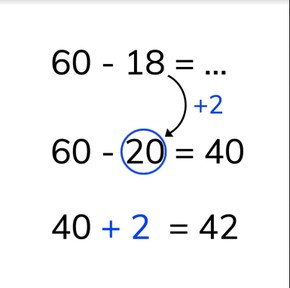Subtraction to 100 via compensation method

# Subtraction to 100 via compensation method8,000 schools use Gynzy92,000 teachers use Gynzy1,600,000 students use Gynzy

## General

Students learn, when subtracting numbers to 100 to use the compensation method, whereby they round one of the numbers to the nearest tens number and then to add what they rounded to the difference.

2.NBT.B.5

## Relevance

It is important to be able to do this so that students are able to easily calculate more difficult subtraction problems.

## Introduction

Remind students that tens are a group of ten and that tens numbers end with a zero in the ones place. Ask students if they can name any more tens numbers. Next have students practice rounding numbers to the nearest ten. The interactive whiteboard has a selection of flags with numbers on them. Take a ball and throw it to a student- that student must round the number you point at. 17 becomes 20, 29 becomes 30 and so on until all the numbers have been rounded.

## Development

Explain to students how to use the compensation method on subtraction problems to 100. A problem like 60 - 18 can be solved easily when you compensate the numbers. Select the number that is closest to a tens number and round it to the nearest ten. The number 18 is rounded to 20 by adding 2 more. The rounded subtraction becomes 60 - 20 = 40. The two you added to 18 are now added to the difference, so 40 + 2 = 42. The difference is the same. Explain to students that sometimes you round the first number, and sometimes you round the second number in the subtraction problem- they must look carefully to see which number is closest to a tens number. Check that students are able to determine which rounded subtraction problem matches a given subtraction problem. You can erase the grey boxes to check their answers. Using the number line, show that you must always look carefully to see which number to round. Ask students to solve the next problems and tell them that they must use a rounded subtraction problem to solve. They can start by writing it out and them move to doing that in their heads.

Check that students are able to use the compensation strategy for subtracting numbers to 100 by asking them the following questions:
- Round the following numbers to their nearest tens number: 27 - 52 - 48 - 79 - 81
- How do you round a number?
- How can you easily solve 73 - 19?
- Which step can you not forget when solving by rounding? (what you have added to a number, must be added to the difference).

## Guided practice

Students must first determine which rounded subtraction problem would help solve the given equation. Then students are asked to use rounding to solve the next subtraction problems. Encourage students to think carefully about what they round, and that they need to add that to the differences.

## Closing

Show the statements of the two students on the interactive whiteboard and ask your class to determine which student is correct. Students must determine which rounded subtraction problem is best. Ask them to explain their thinking. Remind students that it is useful to use the compensation method so that they can round their problems to make subtraction easier. But that they must remember to add to the difference what they added t the number.

## Teaching tips

For students who have difficulty with this learning goal, you can help visualize the rounding by using MAB blocks or other manipulatives. They can then easily see what rounds to a ten and where the blocks move to. Students who are ready to be challenged can be encouraged to do the rounding in their heads.

## Instruction materials

Ball
Optional: MAB blocks or other manipulatives

### The online teaching platform for interactive whiteboards and displays in schools

• Save time building lessons

• Manage the classroom more efficiently

• Increase student engagement